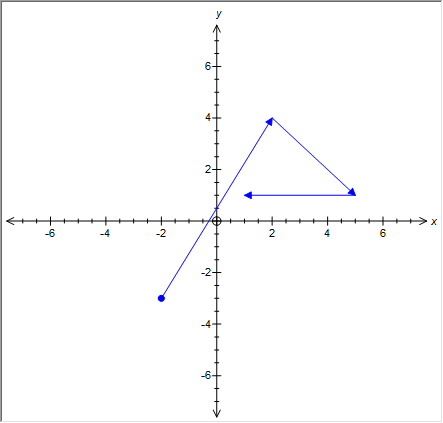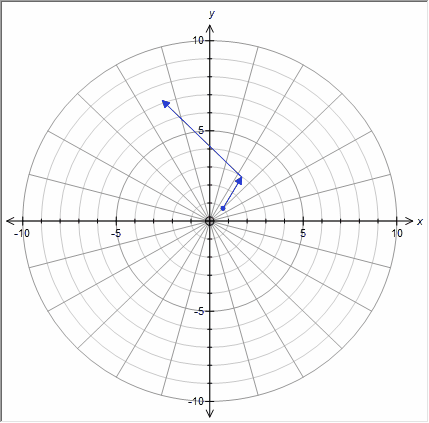﻿ Vectors

# Vectors

Top  Previous  Next
 Vectors are entered as <2,3>.  They can be added, subtracted and combined with a point.  See regional issues   For example:   (-2,-3) + <4,7> + <3,-3> - <4,0>Polar Vectors If you prefix one of the vectors/points with a P, the graph tool will interpret the points and vectors as polars.  You must enter any angles using the current angle measurement (degrees or radians).   p(1,pi) + <2,pi/3> - <6,-pi/4>Constants   Vectors can contain constants which are discussed later in this manual.  You can enter a vector of <-3a,b> for example.     Regional Issues   If you use a comma as a decimal point (eg 3,2) you must use a semicolon to separate the two coordinates.   (-2;-3) + <4;7> + <3;-3> - <4;0>